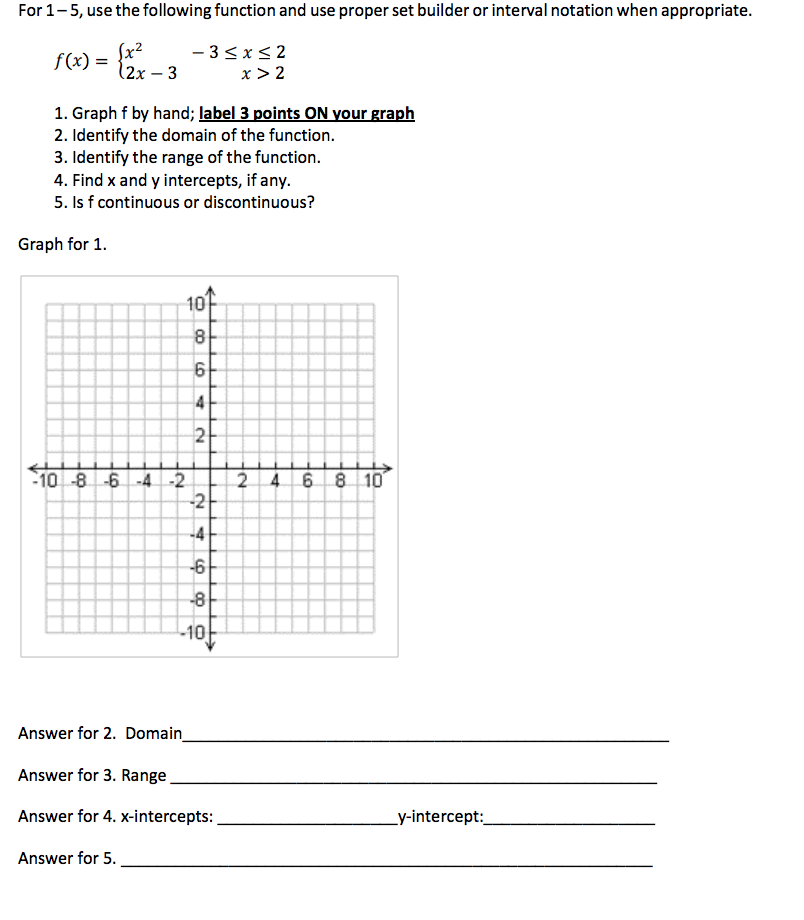# For 1-5, use the following function and use proper set builder or interval notation when appropriatex2sxs22x- 3s(x)r > 21. Graph f by hand; label 3 points ON your graph2. Identify the domain of the function.3. Identify the range of the function.4. Find x and y intercepts, if any.5. Is f continuous or discontinuous?Graph for 110-810 -84 -22 468 12-610Answer for 2. DomainAnswer for 3. RangeAnswer for 4. x-interceptsAnswer for 5.y-intercept:

Question

Can you solve all of them?help_outlineImage TranscriptioncloseFor 1-5, use the following function and use proper set builder or interval notation when appropriate x2sxs2 2x- 3 s(x) r > 2 1. Graph f by hand; label 3 points ON your graph 2. Identify the domain of the function. 3. Identify the range of the function. 4. Find x and y intercepts, if any. 5. Is f continuous or discontinuous? Graph for 1 10 -8 10 -8 4 -2 2 468 1 2 -6 10 Answer for 2. Domain Answer for 3. Range Answer for 4. x-intercepts Answer for 5. y-intercept: fullscreen
check_circle

Step 1

Hello there! I see that there are five subparts in the given quesiton, I cannot answer all of them according to our policies. I will be answering the first three questions.Kindly make a new request for other remaining questions.

Step 2

Given function f(x) as shown below

Let us find the points on the graph for x = -2,0,2,3,4. we get the following values for f(x) respectively.

Step 3

The points on the graph of f(x) for different x values are tabulated below.

Now we use these points and plot the gra...

### Want to see the full answer?

See Solution

#### Want to see this answer and more?

Solutions are written by subject experts who are available 24/7. Questions are typically answered within 1 hour.*

See Solution
*Response times may vary by subject and question.
Tagged in

### Other